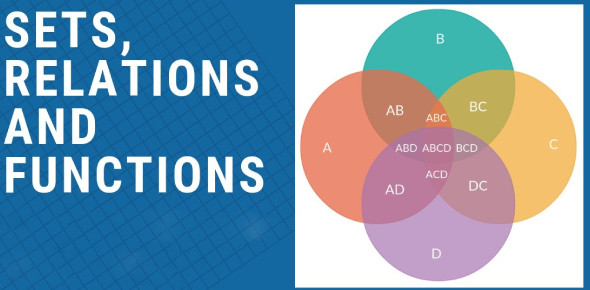# JEE Main Sets Relations And Functions: Quiz!

Approved & Edited by ProProfs Editorial Team
The editorial team at ProProfs Quizzes consists of a select group of subject experts, trivia writers, and quiz masters who have authored over 10,000 quizzes taken by more than 100 million users. This team includes our in-house seasoned quiz moderators and subject matter experts. Our editorial experts, spread across the world, are rigorously trained using our comprehensive guidelines to ensure that you receive the highest quality quizzes.
| By Tanmay Shankar
T
Tanmay Shankar
Community Contributor
Quizzes Created: 547 | Total Attempts: 1,818,423
Questions: 10 | Attempts: 1,713Settings.

• 1.

### If A = {p, q, r, s} and B = {7, 8, 9}, find which of the following is a set of ordered pair, are relations from A to B?

• A.

R = {(7, p), (9, p)}

• B.

R = {(p, 7), (r, 8), (s, 7)}

• C.

R = {(7, s), (8, q), (r, 9)}

• D.

R = {(8, s), (9, q)}

B. R = {(p, 7), (r, 8), (s, 7)}
Explanation
In part (b), the given relation r is a subset of A × B and hence, it is a relation but in all other options, no other relation is a subset of A × B and hence only option (b) is correct.

Rate this question:

• 2.

### In an international conference of 200 people, there are 53 Indian women and 39 Indian men. Of these Indian people, 7 are doctors and 42 are either men or doctors. There are no foreign doctors. How many women doctors are attending the conference?

• A.

1

• B.

2

• C.

3

• D.

4

C. 3
Explanation
Given n (M ∪ D) = 42;
n (M) + n (D) – n (M ∩ D) = n (M ∪ D); 39 + 7 – n (M ∩ D) = 46
n (M ∩ D) = 4;
Amongst the men there are only 4 doctors and as there are no foreign doctors so there are total three Indian women doctors because the total no of doctors is 7.

Rate this question:

• 3.

### In a sports hostel, 21 are on the baseball team, 26 are on the hockey team and 29 are on the football team. 14 play hockey and baseball, 15 play hockey and football, and 8 play all three games. How many total noes of players are there?

• A.

43

• B.

37

• C.

44

• D.

52

A. 43
Explanation
n (B) = 21, n (H) = 26, n (F) = 29;
n (H ∩ B) = 14, n (H ∩ F) = 15, n (F ∩ B) = 12, n (B ∩ H ∩ F) = 8;
Total no of players = n (B ∪ H ∪ F);
n (B ∪ H ∪ F) = n (B) + n (H) + n (F) – n (H ∩ B) – n (H ∩ F) – n (F ∩ B) + n (B ∩ H ∩ F);
n (B ∪ H ∪ F) = 21 +26 +29 – 14 – 15 – 12 + 8 = 76 – 41 + 8;
n (B ∪ H ∪ F) = 43

Rate this question:

• 4.
• A.

{ α∶ π/2≤α≤5π/6}

• B.

{ α∶ π≤α≤3π/2}

• C.

{α∶π/2≤α≤3π/2}

• D.

Both a & b

D. Both a & b
• 5.

### Which of the following is correct about the above functions?

• A.

F is a one-one onto function

• B.

Z = r (cotθ + tanθ), so |z| = r

• C.

F is a one-one but not onto function

• D.

None of these

C. F is a one-one but not onto function
Explanation
clearly f is a one-one function but not onto function f will always be a real number and even numbers do not have any pre-image.

Rate this question:

• 6.
• A.

S = qr

• B.

R = qs

• C.

Q = rs

• D.

None of these

A. S = qr
Explanation
Given that q and r are relatively prime, therefore the given relation: qN ∩ rN = sN can be written as (qr) N = sN which implies that:
s = qr.

Rate this question:

• 7.
• A.

{x : 2 ≤ x < 4 }

• B.

{x : 2 < x ≤4 }

• C.

{x : 2 ≤ x ≤ 4 }

• D.

{x : 2 > x < 4 }

A. {x : 2 ≤ x < 4 }
Explanation

Rate this question:

• 8.
C.
• 9.

### Which of the following is the empty set?

B.
Explanation
From the question it is clearly evident that option (b) is correct as x ∈ R but in the given condition the x belongs to the imaginary values as x = ± 3i.

Rate this question:

• 10.

### Two finite sets have ‘x’ & ‘y’ elements. The total number of subsets of the first set is 56 more than the total number of the second set. Which of the following are the values of ‘x’ & ‘y’?

• A.

7, 6

• B.

5, 1

• C.

8, 7

• D.

6, 3Back to top